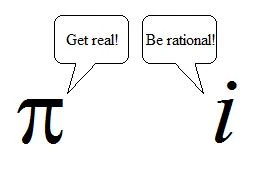## FANDOM

37 Pages

Number systems are numbers grouped in specific areas of mathematics. All numbers we work with, both real and imaginary, are complex.

Complex numbers are divided into real and imaginary numbers. Real numbers then branch out into rational and irrational numbers. Rational numbers branch into integers, then integers branch into whole numbers, and whole numbers branch into natural numbers. This page will discuss all categories aforementioned.

## Real and Imaginary NumbersEditIn a coordinate plane, think of the x-axis representing real numbers and the y-axis representing imaginary numbers.

### Real NumbersEdit

Real numbers are numbers that do not involve i, the function for imaginary numbers. Examples of real numbers are 3, -8, e, and $\pi\,$.

### Imaginary NumbersEdit

Imaginary numbers involve integers and i.

$\sqrt{-3}\, = i\sqrt{3}\,$
$\frac{i}{2i}\, = \frac{1}{2}\,i$
$i = \sqrt{-1}\,$

Imaginary numbers cannot be broken down any further, so it is its own little stub of a branch on the tree of complex numbers. How sad.

## Rational and Irrational NumbersEditA clever joke involving $\pi\,$ and i.

### Rational NumbersEdit

Rational numbers either repeat or terminate in decimals. They can each be expressed as a fraction.

$\frac{1}{\infty\,}\, = 0.\bar{0}\,1$
$\frac{1}{7}\, = 0.142857142857142857142857142857...$
$\sqrt{-216}\, = -6$
$\sqrt{216}\, = 6$

### Irrational NumbersEdit

Irrational numbers neither repeat nor terminate. They cannot be expressed as a fraction. Most of the real numbers are irrational.

$\pi\, = 3.141592653589793238462643383279502884197...$
$\sqrt{5}\,$

Irrational numbers cannot be broken down any further, so it is its own branch of the complex number tree. Again, how sad.

## IntegersEdit

Refer to the coordinate plane. For integers, imagine the x-axis alone. That is a number line, the best way to depict integers.

Integers include negative numbers, zero, and positive numbers.

$..., -3, -2, -1, 0, 1, 2, 3,...$

## Whole NumbersEdit

Whole numbers do not include negative numbers. Sorry, $-\infty\,$.

$0, 1, 2, 3, 4,...,\infty\,-1, \infty\,$

## Natural NumbersEdit

Natural numbers are the numbers you usually count with. Zero is omitted in the natural number system. Sorry, 0.

$1, 2, 3, 4,...\infty\,-1, \infty\,$

## See AlsoEdit

Community content is available under CC-BY-SA unless otherwise noted.